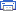# Inductive meter adapter Electronic Schematics > Test and measurement > Inductive meter adapter

The following circuit enables me to measure inductance of the inductor labeled LX which is the inductance to be measured. The o/p of the circuit is a TTL square wave whose frequency relates to the inductance being measured.
The inductance meter adapter output is connected to a frequency meter and the inductance is calculated from the frequency. Hence you need a frequency meter and some calculation to get your inductor value.

HOW IT WORKS
The heart of the circuit is the buffer colpitts oscillator(the first stage) which resonates with the unknown inductance to give a  Sine wave of a particular frequency . The frequency of the sine wave is a function of the unknown inductance and the four 1000pF capacitors. The output sine wave is amplified by the second transistor and is then rectified by the capacitor and diode combination that follows. The rectified sine wave now having only positive excursions is buffered by the third transistor and is then fed to the 74ls393  Counter ic which is configured as a divide by 256 counter. The output of the ic pin 6 and ground is connected to the frequency meter.

NOTES
The 7805 regulator powers the IC and the last 2 transistors
The circuit operates from a 9V battery which also feeds the regulator
The Ic consists of two counters in one package of 14 pins hence you need just one.
PIN 14 is VCC and PIN 7 is ground
The ic is TTl IC

My own digital meter can read frequency so I use it to take the reading.
I programmed my calculator to get me the inductance value from the reading. A simple computer programme can do this as well.
Actual frequency=1/(2**(Lx(uH)*1000e-12))
So the last equation allows you to solve for the value of the inductance Lx which will be in microhenries.

This inductance measuring scheme works well for inductances of even low values less than 1uH. However the lower
Values could have error due to the circuit including test lead inductance. The circuit is that sensitive. I play with some tricks to get around this which I will discuss later if people are interested in the circuit. I wish it was a stand alone unit but here in my country NIGERIA(IN WEST AFRICA) and my city Ibadan there are no fancy PICS or Digital displays to make it a stand alone unit so I just have to make do with Simple IC’S and transistors.

Sorry the equations don’t look too neat I hope you get them though.
And possibly make a stand alone unit with microprocessors or PICS and displays that I haven’t got.

All resistances are in ohms
7805 positive regulator 5v o/p
2SC9018 is a high frequency transistor with FT of 1.1 Ghz . you need high frequency transistors in the circuit where it is used in case you want to substitute for it. I guess 2n2857, 2n5179 ,BF180 can be substituted

By Tosin Osanyintuyi (TAFRICA)
tosinopro@yahoo.com

Print version: